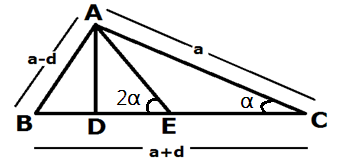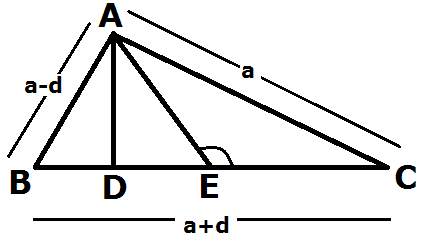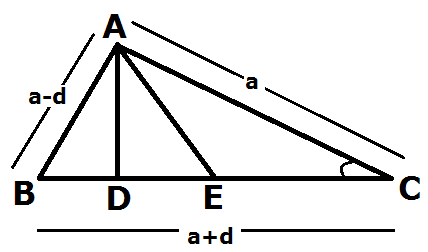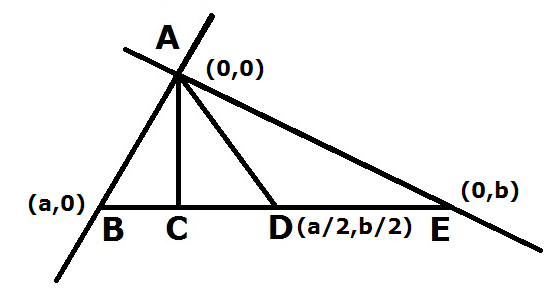EN9k2k09k

For our ancestors who didn’t have the benefit of a world map...

10 min ago
edit
delete

For our ancestors who didn’t have the benefit of a world map...

Rizom - April,2018For our ancestors who didn’t have the benefit of a world map...

Rizom - April,2018For our ancestors who didn’t have the benefit of a world map...

Rizom - April,2018100488
PK 2017-11-23 10:33100488
Piyush Theorem with Four Proofs.....

### A New Theorem introduced by Piyush Goel with Four Proofs

Mathematics for Piyush is a Passion from his childhood he was so passionate about Mathematics used to play with Numbers draw figures and try to get sides distance one day I draw a AP SERIES Right Angle Triangle( thinking that the distance between the point of intersection of median & altitude at the base must be sum of rest sides that was in My Mind).

And at last Piyush Succeed. This new Theorem proved with Four Proof(Trigonometry/Co-ordinates Geometry/Acute Theorem/Obtuse Theorem).

Here are the Proofs:

This Theorem applies in Two Conditions:

1. The Triangle must be Right-Angled.
2. Its Sides are in A.P. Series.
1. Proof with Trigonometry
1. Proof with Obtuse Triangle Theorem
1. Proof with Acute Triangle Theorem
2. Proof with Co-ordinates Geometry

Four Proof ( TRIGONOMETRY/CO-ORDINATES/OBTUSE TRIANGLE/ACUTE TRIANGLE)

(By PIYUSH GOEL)

Theorem: In a Right-Angled Triangle with sides in A.P

Theorem: In a Right-Angled Triangle with sides in A.P. Series, the distance between the point of intersection of median & altitude at the base is 1/10 Th the sum of other two sides.

This Theorem applies in Two Conditions:

1. The Triangle must be Right-Angled.
2. Its Sides are in A.P. Series.

• Proof with TrigonometryAD= DE tan 2 α   —————-2

DC tan  α = DE tan 2α

(DE+EC)  tan  α = DE tan 2α

DE tan  α  + EC tan  α = DE tan 2α

DE tan  α  + EC tan  α = 2 DE tan  α / (1- tan^2  α)

DE tan  α   – DE tan^3  α + EC tan  α –EC tan^3  α  = 2 DE tan  α

EC tan  α –EC tan^3  α- DE tan^3  α = 2 DE tan  α – DE tan  α

tan  α (EC – EC tan^2  α – DE t an^2  α )= DE tan  α

DE tan^2  α  – DE = EC tan^2  α  – EC

-DE ( tan^2  α + 1) = -EC (1 – tan^2  α )

DE (sin^2 α  /cos^2 α + 1) = EC (1- sin^2 α  /cos^2 α )

DE (sin^2 α + cos^2 α /cos^2 α ) = EC (cos^2 α – sin^2 α /cos^2 α )

DE (sin^2 α  + cos^2 α ) = EC(cos^2 α  -sin^2 α )

DE (sin^2 α  + cos^2 α ) = EC (cos^2 α  -sin^2 α) ……..where (sin^2 α  + cos^2 α =1) & (cos^2 α  -sin^2 α = cos 2 α  )

DE= EC cos 2 α

cos α   =a/a+d   & sin α = (a-d)/ (a +d)

cos^2 α  = a 2/ (a +b) 2

sin^2 α  = (a-d) 2/ (a+ d) 2

DE= EC (cos^2 α    – sin^2 α )

= EC ( a 2 / (a +b) 2 – (a-d) 2/ (a +d) 2

= EC ( a 2 – (a-d) 2/ (a +d) 2

= EC (a –a +d) (a+ a-d)/ (a+ d) 2

= EC (d) (2 a -d)/ (a+ d) 2

= (a +d)/2(d) (2 a -d)/ (a +d) 2 ————- where EC= (a +d)/2

= (d) (2 a -d)/2(a +d)

= (d) (8 d -d)/2(4 d+d)       ——————where a= 4 d (as per the Theorem)

= 7 d 2 /2 (5 d)

= 7 d /10

= (3 d+4 d)/10= (A B+AC)/10

• Proof with Obtuse Triangle TheoremAC 2=EC 2 +A E 2 +2 C E. DE       where EC = (  a +d) /2,A E=( a +d)/2

a 2 = (a +d/2)2 + (a+ d/2)2 + 2(a +d)/2 DE

= (a +d/2) (a+d+2 DE)

= (a +d/2) (a+d+2 DE)   where a= 4 d

16 d 2 = (5 d/2) (5 d+2 DE)

32 d/5 = 5 d + 2 DE

32 d/5 – 5 d = 2 DE

32 d -25 d/5 = 2 DE

DE =7 d /10

= (3 d+4 d)/10 = (A B+AC)/10

• Proof with Acute Triangle TheoremA B 2= AC 2+BC 2 – 2 BC.DC

(a-d) 2= a 2 + (a+ d) 2 -2(a+ d) (DE+EC)         where A B= (a-d), AC=a, BC =( a +d) & EC= (a +d)/2

(a-d) 2 – (a +d)2 = a 2  -2(a +d)(DE+EC)

(a- d –a-d) (a -d +a +d)  = a 2 -2(a+ d) (2 DE+a+d)/2

2(-2 d) (2 a) = 2 a 2 -2(a +d) (2 DE+a+d)

-8 ad – 2 a 2 = -2(a +d) (2 DE+a+d)

-2 a (4 d   +a) = -2(a +d) (2 DE+a+d)

a (4 d  + a) = (a +d)(2 DE+a+d)

4 d (4 d 4 d) = (4 d+d) (2 DE+4 d+d)

4 d (8 d) = (5 d) (2 DE+5 d)

32 d 2/5 d =   (2 DE+5 d)

32 d/5 =   (2 DE+5 d)

32 d/5 – 5 d =   2 DE

(32 d – 25 d)/5 =   2 DE

DE = 7 d/10

= (3 d+4 d)/10 = (AB+AC)/10

1. Proof with Co-ordinates GeometryIn Triangle A B C,point A,B&C,s co-ordinates are respectively (0,0) ,(a,0)&(0,b).

Point D is middle point ,co-ordinates of Point Dis (a/2,b/2)

Equation of BE is ……………… ( Two Points equation)

Y –Y1 =(X – X)(Y2 –Y1)/(X2 – X)

Y – 0 =b-0/0-a(X – a)

Y = -b/a(X) + b——————- (1)

M 1 = -b/a

For perpendicular

M1 M2= -1

M2= -1/M1
So M2= a/b

Equation of AC

Y – 0 = a/b(X-0)

Y=a/b(X) —————— (2)

Put Y value in equation (1)

a/b(X) + b/a(X) =b

X (a 2+b 2/a b) = b

X = a b 2/ (a 2 + b 2)

To get Value of Y, put X value in equation (2)

Y = a/b (a b 2/ (a 2+b 2)

Y = a 2 b/ (a 2+b 2)

Here we got co-ordinates of Point C – a b 2/ (a 2 + b 2), a 2 b/ (a 2+b 2) and co-ordinates of point D is (a/2, b/2) because d is midpoint.

As per the A.P Series (z-d,z,z+ d)

Hers a= z-d, b= z, c = z+ d

(z +d) 2= (z-d) 2+ z 2

(z +d) 2- (z-d) 2 = z 2

(z +d +z-d)(z +d – z + d) = z 2

(2 z)(2 d) = z 2

4 z d = z 2

4 d = z

Put value of a & b

a b 2/ (a 2 + b 2), a 2 b/ (a 2+ b 2) & (a/2, b/2)

a b 2/ ( a 2 + b 2) = 48 d/25

a 2 b/ (a 2+b 2) = 36 d /25

a/ 2=3 d/2

b/ 2 =4 d/2

CD 2= (48 d/25 -3 d/2)2-(3 6 d/25-4 d/2)2

= (96 d-75 d/50)2 + (72 d-100 d/50)2

= (21 d/50)2 + (-28 d/50)2

= (441 d 2/2500) + (784 d 2/2500)

= (1225 d 2/2500)

CD= 35 d/50 = 7 d/10

= 7 d/10 = (3 d+4 d)/10 = (A B+A E)/10

written by

PK